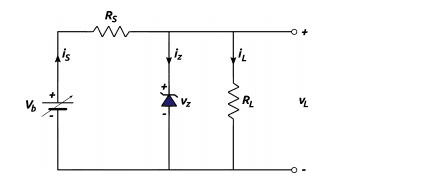# Zener diode circuit-variable voltage source

• Engineering
• Rampart

## Homework Statement

This diode zener has breakdown voltage equal to Vz=8.2V for currents: 75mA<=iz<=1A.If RL=9 Ω,how much must Rs be,so that VL=Vz=8.2V,while Vb changes at about _+10% in relation with the nomimal operating current,displayed in the data sheet which is 12V?## The Attempt at a Solution

It is located in the pdf below.Also i have to apologize if the exercise wasn't totally understood,but i did my best in order to translate it from my language.Thank you in advance for your time and effort.

#### Attachments

• New Doc 31.pdf
1.6 MB · Views: 328

Do a check of your results. The load current ##I_L## is fixed at:

##I_L = \frac{V_z}{R_L} = \frac{8.2 V}{9\Omega} = 0.911 A##.

If ##V_b## is at its maximum of 13.2 V and ##R_s## is at your minimum value of 1.3612 Ω then the supply current is

##i_s = \frac{13.2 V - 8.2 V}{1.3612 Ω} = 3.673 A##

which means that the zener needs to conduct ##i_s - I_L = 2.762 A## , exceeding the zener's maximum current of 1 A.

At first let me thank you sir for your reply.Now as far as the exercise is concerned yes the numbers aren't right if Rs=1,3612Ω and Vb=13.2V but who said they were.I just found the set for Rs.Of course the numbers cannot be right for each element of the set Rs for each element of the set Vb.But surely there is an element in the set of Rs,which in combination with at least one element in the set of Vb, gives me the right numbers.
Or
Have i understood wrong the exercise?Maybe i am asked to find a set for Rs(or a specific number) with which,for every Vb that belongs to the set Vb,the numbers must be always right?Because if that is the case then,well dang :P

In a practical situation the idea would be to find a value (or range of values) for ##R_s## that would be safe for the full range of ##V_b##. That is the approach that I would advocate.

Then this exercise got to a new level.I don't believe i can find the right range by substituting or by approaching values and trying.I must be missing a relation or sth.Anyway i will give it some thought.Thank you again sir.

Hint:

Suppose you were to find an allowed range of values for Rs when Vb is at its low value: What is Rs if Iz is 0.75 A? What is it if Iz is 1 A? Since that's the allowed range for the zener current, the zener would be safe under low voltage conditions with Rs in that range.

Do the same for when Vb is at its high value. Do the two ranges for Rs overlap?

Yes sir they do.I found 2,6178<=Rs<=2,6399.Actually it is not the right right answer as some values are missing, because of the approaches i took to the numbers in order to help myself.But it is somewhere there :)

Hint:

Suppose you were to find an allowed range of values for Rs when Vb is at its low value: What is Rs if Iz is 0.75 A? What is it if Iz is 1 A? Since that's the allowed range for the zener current, the zener would be safe under low voltage conditions with Rs in that range.

Do the same for when Vb is at its high value. Do the two ranges for Rs overlap?
Correction: line 1 should read "... if Iz is 0.075A."

Correction: line 1 should read "... if Iz is 0.075A."
Whoops. You're right. My bad